This video was brought to you by GeneralPAC.com, making power systems Intuitive, Open and Free for Everyone, Everywhere. Consider subscribing and supporting through patreon.com/GeneralPAC. This is a mechanism for you to support us financially so we can continue making high quality power system video tutorials. Our corporate sponsor for this topic is AllumiaX.com from Seattle, Washington. Contact them for industrial and commercial power system studies.

A tutorial on the Newton Raphson Power Flow example. This is part two of the tutorial. Remember, that in this tutorial we just took an example from Granger and Stevenson's book and this is example number 9.3 of the book in section 9.4. This example is so general that hopefully you'll get a much better understanding of the Newton Raphson method.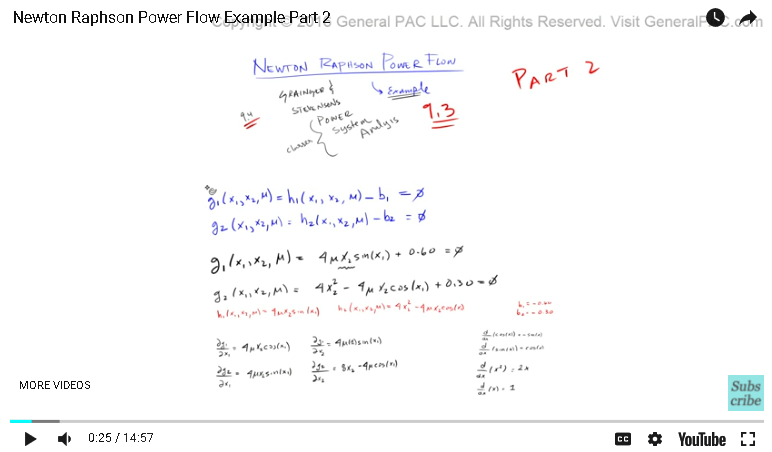Now, again in this tutorial we're only gonna be concerned about the actual method, we won't really step into the theoretical realm. Okay. We left off here, and as you can see we kind of worked our way from the definition of the equations, and then how the equations were presented in the book. And then, we did our partial derivatives to form our Jacobian matrix.

Now, these are all the partial derivatives of G1 and G2, respectively. When we say that we have the basic building blocks of the Jacobian matrix, what do we mean by that? Well, the Jacobian matrix is a fancy word of just saying a matrix of the partial differential equations.

In our example, the Jacobian matrix J, that is equal to DG1 to DX1, DG1 to DX2, DG2 to DX1, and DG2 to DX2. This right here is our Jacobian matrix. Not too bad. Right? We've already solved the partial derivatives here, so the Jacobian matrix simply equals 4UX2 cosign of X1, 4U sign of X1, 4U sign of X1, and 8X2. Here is the result. Again, we're still in this realm of the Jacobian matrix.

Now, let's just make this a little bit smaller so we have some working space. Now, let's just recap and talk about some of the variables that we already have and introduce some of our initial variables. Okay? First off, one of our initial variable ... We'll call this X1. We'll just place a star here, just to distinguish it from everything else. The X1 is equal to 0, that's one of our initial variables. X2, the other initial variable, that is equal to one.

We're gonna say that the other initial variable, which is just a U, that is also equal to one. Remember, we've already figured out what our B1 and B2s were. B1 is equal to -0.60 and B2 is equal to -0.30. Okay. These are our initial values. Okay? Now, the next step is we're going to find out what our initial mismatch is.

In here, we're not really going to go over what these terms mean. Not yet anyways. We'll first introduce the hand calculation part, so that we get an overall understanding of how this process works. And then, we'll go into detail a little more later.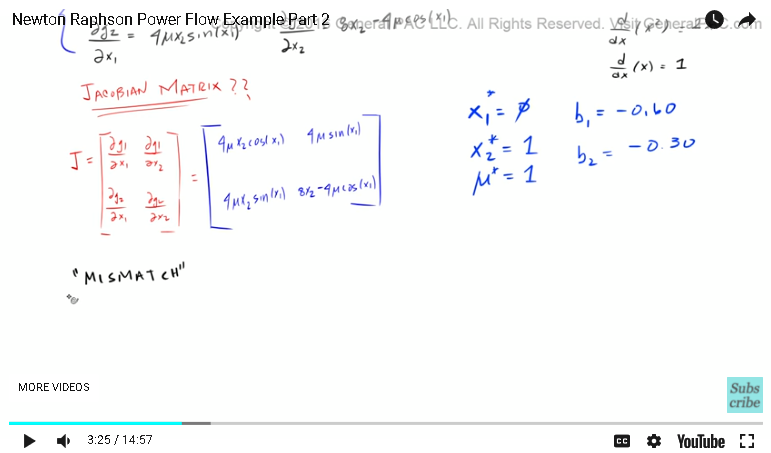The mismatch is equal to ... Well, the first mismatch is delta G1. Okay? We're gonna say that this is the zero-ith iteration and that is equal to B1 minus H1 of the zero-ith iteration. In H1, we're going to feed it the initial values that we came up with. Remember, these are the initial values. I'm gonna ... Similarly, we're going to have a delta G2, the zero-ith iteration. That's going to equal B2 minus H2, the zero-ith iteration.

Same thing here. We're gonna feed it initial values or initial conditions. Now, let's actually solve this guy. Remember, H1 was ... We've already said what H1 was. Well, H1 is this term right here, which comes from this particular equation. H1 is simply equal to 4UX2 times sign of X1. H2 is equal to 4X2 squared minus 4UX2 times cosign of X1. All we need to do is we just need to plug and chug. Right? Plug in our initial values into these two equations and we'll have our initial mismatch.

We already know what B1 was. Well, B1 is just -0.60. We're gonna say that B1 is equal to -0.610 minus H1. Now, H1 was 4U, sign of X1. What does that equal? Well, now that's pretty straight forward. Right? Because, we know what U1 was. Well, U1 we set that equal to one. We know what X2 is, we set X2 to equal one. Right? Right here, look at this right there. We know what X1 is, X1 was set to equal zero. Now we have four times one times one times the sign of zero.

Now, the sign term, I can never remember if it's a zero or a one. I always have to draw this unity circle out. We're talking about this point right here. That point is 0-1 in the coordinate system and this represents the coordinates for the Y axis, and this represents the coordinates for the X axis. Again, this is sign and this is cosign.

We know that the sign of zero is equal to zero. Right? Sign of zero is equal to zero. This entire term becomes -.60 minus zero and that is equal to just -.60. And then, this term right here, let's equate that. That is equal ... Well, we know what B2 is. B2 was -0.30, so -0.30 minus H2. Now, H2 was this term right here. 4X2 squared minus 4UX2 times cosign of X1. That is equal to 4X2 squared minus 4UX2 times cosign of X1. Okay? What does that equal?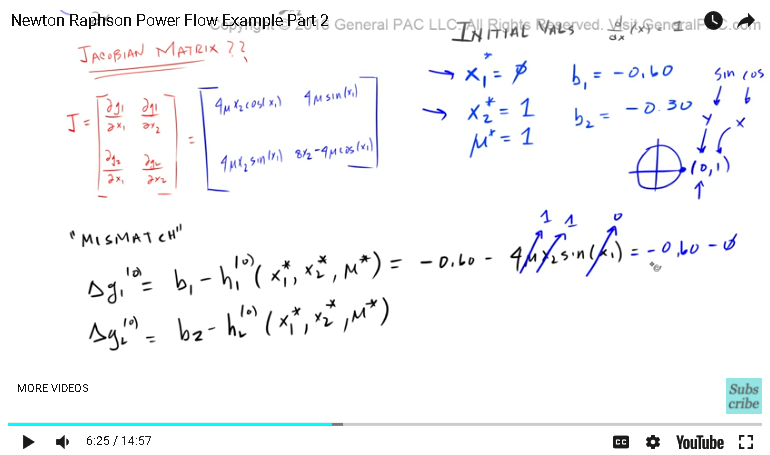Now, same exact procedure. Right? We know what 4X2 is, 4X2 is just equal to one. And then, we know what U is equal to, U is equal to just one. We know what X2 is equal to, X2 is equal to also one. And then, X1 is equal to zero. Now, the cosign of zero should equal one. Right? That entire equation becomes -0.30, which represents this B2. Minus ... And then, we'll have H2 in this bracket here. Hopefully that's big enough.

The H2 term is this term right here. Right? First, we have this 4X squared. We know that is equal to just four minus this term right here. Now, four times ones times one times cosign of zero is one. Minus that by four ... Turns out this was too big of a bracket. We know that four minus four is just equal to zero, that term becomes 0.30. Okay. Cool.

Now, let's just clean this up. Awesome. Now, let's make this a bit smaller. Okay. Now that we've calculated this mismatch, let's now use the same initial equations to calculate each element of the Jacobian matrix. The first element was DG1 over DX1 and we're going to give it our initial conditions. That is going to equal ... Well, let's figure out what this DG1 over X1 equals.

The partial derivative of DG1 over DX1, that is equal to four times U times X2 times the cosign of X1. That is equal to 4UX2 times the cosign of X1 and we know that, that's equal to four. We know what U is, U is equal to one. We know what X2 is, X2 is also equal to one. Right? These are our initial variables. We know what X1 is, but the cosign of X1. Which is X1 is equal to zero, so the cosign of zero is equal to one. Okay? This partial derivative then just becomes four. Okay.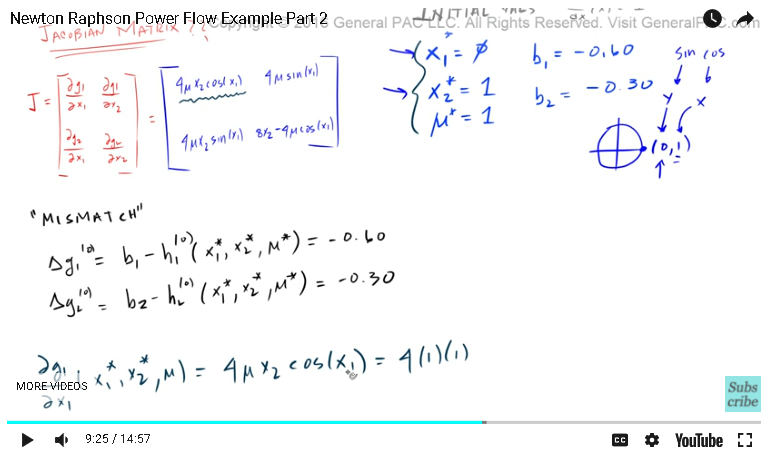Now, let's move onto our next partial derivative, which is DG1 over DX2. We're gonna be inputting the same initial conditions, which is just 011 and that is equal to ... Again, DG1 over DX2 is this guy right here, which corresponds to this right there. That is equal to four times U times sign of X1. That, my friends, are equal to four times U ... Which, U is equal to one times the sign of X1 ... Excuse me. The sign of zero is equal to zero. Put a zero there so this entire term becomes zero. Okay.

Now, let's go to our next partial derivative. The partial derivative G2 with the resect to DX1. G2 with respect to DX1 was this right there, which is equal to this there. That's 4UX2 times sign of X1. We're gonna give it this initial conditions, four times U times X2 times the sign of X1 and that is gonna equal ... Four times U is just one. X2 is one, X1 is zero. The sign of zero is going to equal zero. Right there. The sign of zero is equal to zero. That is also equal to zero there.

Alright. The next partial derivative is this, DG2 over DX2. Again, we're gonna feed it the same initial conditions. That derivative is equal to this guy right here. 8X2 minus ... I think there's a square there? No. There isn't. 8X2 minus 4U times cosign of X1. 8X2 minus 4U, cosign of X1. Okay? Now, that is equal to eight times X2 ... X2 was this term right here. It's eight times one, which is just eight. Minus four times ... U is one. Times the cosign of X1.

Now, the cosign of X1 was just one. Right? Cosign of X1 is just one, so that becomes four times one times one and that is equal to four. Okay. Now, let's make this guy really small. Okay. We have our partial derivatives for the zero-ith iteration. I forgot to mention that this guy ... Let's zoom in here. These guys are for our zero-ith iteration, so our partial derivative for the zero-ith iteration. Okay.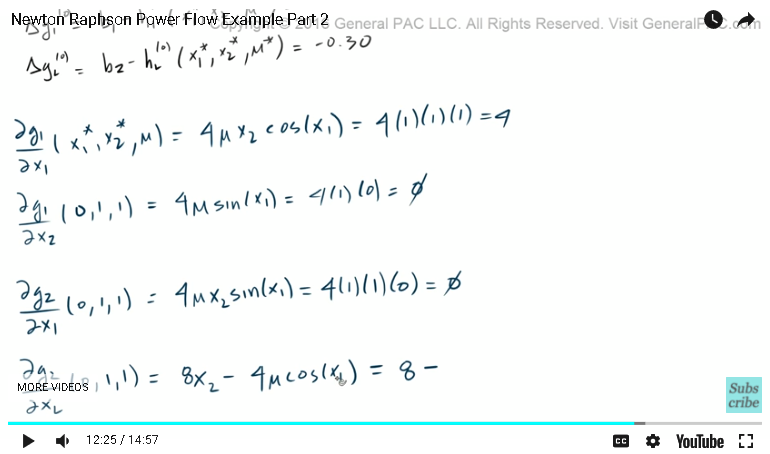Which means, that the Jacobian matrix ... The zero-ith iteration of the Jacobian matrix, that's going to equal the partial derivative. That's gonna equal this guy right there. Okay? The Jacobian matrix for the zero-ith iteration is the partial derivative of G1 with the respect of X1 for the zero-ith iteration and G1 with the respect of X2. DG2 with respect to X1, DG2 with respect to X2, which is this term right here. Which, is gonna be four.

And then, this term DG1 over DX2, which is this guy right here. DG1 over DX2, that's gonna equal to zero, so this right here is zero. And then, DG2 over DX1, DG2 over DX1. That's gonna equal zero. DG2 over DX2, DG2 over DX2. That's gonna equal four. Alright. This is looking pretty darn good. Now, let's make this guy small.

Alright. Before we continue on to part three, if you haven't already, please click on the bottom right corner of the screen and subscribe. If you have questions, there's a link on the bottom of this video to the Q&A forum. Just click on that link and ask away. Lastly, this video was brought to you by GeneralPAC.com, "Making Power Systems Intuitive." Thank you.

#### Greetings from the GeneralPAC Team!

We make high-quality Power Systems Video Tutorials on complex topics that are free and open to everyone!  Thank you so much for supporting us through Patreon so we can continue doing good and valuable work.

What is Patreon and why do we use it?

Patreon is a fantastic portal that allows our fans and community to make monthly contribution (like Netflix subscription) so we can continue creating high-quality power systems video tutorials. In return, you get access to incredible perks like voting on future topics, getting your questions answered, access to VIP Q/A webinars with the creators of GeneralPAC, and much more! We THANK YOU for supporting us

Why do we need your support?

An incredible amount of time and effort is needed to develop high-quality video tutorials. Each video (Part 1 for example) takes approximately 10 hours to complete which includes learning the concept ourselves, brainstorming creative ways to teach and explain the concepts, writing the script, audio recording, video recording, and editing. It's no wonder why Hundreds-of-Thousands of people have watched, liked, subscribed, and left positive comments on Youtube channel. Your support truly makes all the difference.